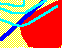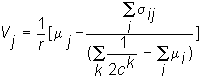7.17 CAPITAL STRUCTURE IMPLICATIONS

Recall from the construction of equilibrium that the CAPM equation for the value of the firm is:This valuation model computes the present value of risk-adjusted cash flows discounting at the risk-free rate of interest. You can see, however, that the nature of the financial claim on the firm (i.e., stock or bond) does not enter into this equation. That is, if total cash flow projections for the firm, the mi(s), are independent of whether stocks or bonds have been issued, then the market value of the firm is independent of how the firm has financed its assets. That is, the value of the firm is independent of the firm's capital structure. The key assumption here is that projected cash flows are not contingent upon the way that the firm is financed.

For the case of perfect markets, we can illustrate that capital structure is irrelevant, in a simple example in which the firm can issue stocks or bonds. We show that if the bonds are risk-free (i.e., the face value of the debt issue is covered in every state of the world) investors can "re-engineer" any desired capital structure personally by forming a portfolio of risk-free bonds and risky stock. This is discussed in the following example of risk-free firm debt.

previous topic

next topic